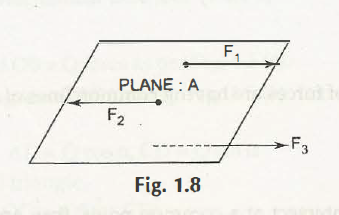Coplanar Parallel Forces can be explained when the forces act on the same plane and also they are parallel to each other.Here, in the picture there are three different forces F1, F2 and F3. They act on the same plane A, but points of forces are different than each other, however they act parallel to each other.

Some important points when a person points out the fact of coplanar Parallel –

• These are parallel forces and thus these will not intersect at any particular point.
• The forces act in a parallel way and thus parallelogram is not perfect to find out the resultant of two parallel forces.
• The resultant of such forces can be evaluated through the “Principle of Moments.”

Parallel forces are divided as – like forces and unlike forces. At the moment when the magnitude is same then it is called the like force and when the resultants are not similar, then it is called unlike forces.

Links of Previous Main Topic:-

Links of Next Mechanical Engineering Topics:-

### Submit Your Assignment### Customer Reviews

My Homework Help
Rated 5.0 out of 5 based on 510 customer reviews at